# Electricity Practical Activities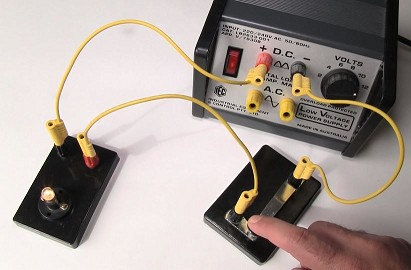The practical activities below will support the content that is covered in the 5-part Shedding Light on Electricity series. This series covers
• how electricity is generated
• electric circuits
• voltage, current, and resistance, and
• electrical safety.

Some ideas for teaching Electricity. This is what I do when I teach electricity to my Year 9’s.
• Ask them to connect a battery, a switch, and a light globe so that the light globe lights up when the switch is pressed. Some know exactly what to do and some don’t, so they might need assistance.
• Ask them to draw the set up (in pictures).
• Tell them to modify the circuit to include a second light globe.
• Tell them to draw the second circuit.
• Explain that pictures take too much time and then introduce circuit symbols: battery, switches, wires, light globes.
• Tell them to redraw their pictures using only circuit symbols (and to never draw pictures again!!).
• complete the first two pracs below. (The third “extension” prac can be done by the students who finish pracs 1 and 2.)
Depending on the timetable, I will intersperse the pracs with the Shedding Light on Electricity series.

(These two videos are 20 seconds and 44 seconds long respectively.)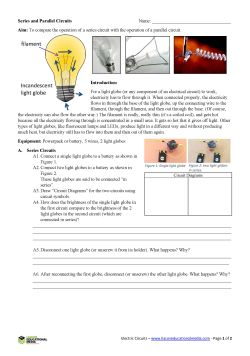An Introduction to Series and Parallel Circuits. In this practical activity, students investigate series and parallel circuits. Beware of the short circuits though!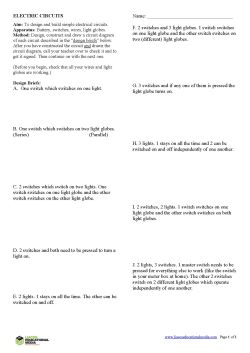Designing Electric Circuits (pdf)  In this practical activity, which requires plenty of higher-order thinking, students have to design, construct, and draw up various electrical circuits according to set design briefs.
By signing each circuit as it is completed, teachers can ensure that the students have actually created the circuit and that they haven’t just copied from another group! See the Electric Circuits Prac video above.

Electric Circuits Extension Activities (pdf) is similar to the prac above but the circuits require two-way switches (see the Extension Electric Circuits video above). I generally finish up after a majority of students have finished the first two pracs. Typically, about half the groups within the class begin the “Extension Activities” sheet and complete some of the circuits by the time the rest have finished the “Electric Circuits” prac.
All of the above typically takes about 6 or so 45-minute lessons.

Once they’ve actually built the circuits in real life, I introduce the students to this simulation: https://sciencedemos.org.uk/electronics.php. I then give them a period to “build” the circuits in the virtual environment. The simulator is free and it’s really good!!

(The video goes for 1 minute and 48 seconds.)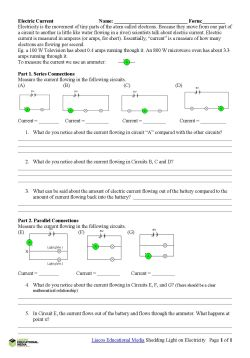Electric Current and Ammeters Practical Activity (pdf). In this activity, students learn to use ammeters and learn the basics of how electric current flows in series and parallel circuits.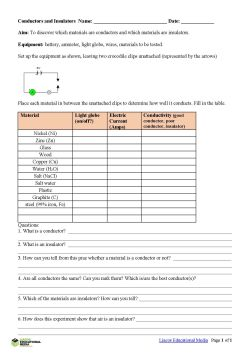Conductors and Insulators Practical Activity (pdf). In this activity, students become familiar with conductors and insulators.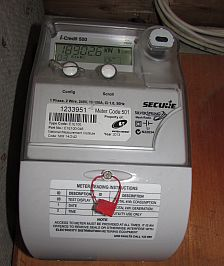The following activities are all about electricity usage in the home.

Electricity Usage. In this task students are asked to find out how much electricity they use over a period of a few days and to calculate the cost of that electricity. Some practice tasks are included on Page 2. For instructions on how to read an older-style meter (the ones that have the dials), click this link to access Energylinx’s explanation. The newer “Smart Meters” are easy to read. Simply push the “Scroll” button to cycle through the display until you get to the display that says “kW h”. The meter in the picture says 18902.6 kW h. This is the total amount of kilowatt-hours that have been used since the meter was installed.

The Kilowatt-Hour. This worksheet allows students to calculate the energy use of a range of common appliances and the cost involved.The Shedding Light on Electricity Series
Shedding Light On Electricity Overview